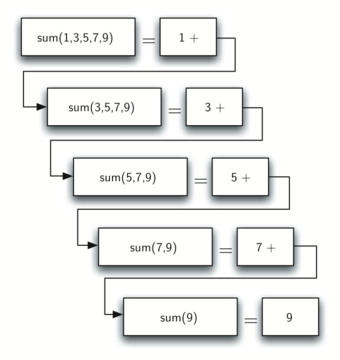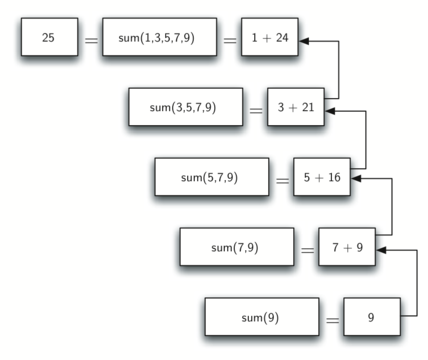# 5.3. Calculating the Sum of a Vector of Numbers¶

We will begin our investigation with a simple problem that you already know how to solve without using recursion. Suppose that you want to calculate the sum of a vector of numbers such as: $$[1, 3, 5, 7, 9]$$. An iterative function that computes the sum is shown in ActiveCode 1. The function uses an accumulator variable (theSum) to compute a running total of all the numbers in the vector by starting with $$0$$ and adding each number in the vector.

Pretend for a minute that you do not have while loops or for loops. How would you compute the sum of a vector of numbers? If you were a mathematician you might start by recalling that addition is a function that is defined for two parameters, a pair of numbers. To redefine the problem from adding a vector to adding pairs of numbers, we could rewrite the vector as a fully parenthesized expression. Such an expression looks like this:

$((((1 + 3) + 5) + 7) + 9)$

We can also parenthesize the expression the other way around,

$(1 + (3 + (5 + (7 + 9))))$

Notice that the innermost set of parentheses, $$(7 + 9)$$, is a problem that we can solve without a loop or any special constructs. In fact, we can use the following sequence of simplifications to compute a final sum.

$\begin{split}total = \ (1 + (3 + (5 + (7 + 9)))) \\ total = \ (1 + (3 + (5 + 16))) \\ total = \ (1 + (3 + 21)) \\ total = \ (1 + 24) \\ total = \ 25\end{split}$

How can we take this idea and turn it into a C++ program? First, let’s restate the sum problem in terms of C++ arrays. We might say the sum of the vector numVect is the sum of the first element of the vector (numVect), and the sum of the numbers in the rest of the array (numVect.erase(numVect.begin()+0)).

In this equation $$first(numVect)$$ returns the first element of the array and $$rest(numVect)$$ returns an array of everything but the first element. This is easily expressed in C++ as shown in ActiveCode 2.

There are a few key ideas while using vector to look at. First, on line 6 we are checking to see if the vector is one element long. This check is crucial and is our escape clause from the function. The sum of a vector of length 1 is trivial; it is just the number in the vector. Second, on line 11 our function calls itself! This is the reason that we call the vectsum algorithm recursive. A recursive function is a function that calls itself.

Figure 1 shows the series of recursive calls that are needed to sum the vector $$[1, 3, 5, 7, 9]$$. You should think of this series of calls as a series of simplifications. Each time we make a recursive call we are solving a smaller problem, until we reach the point where the problem cannot get any smaller.Figure 1: Series of Recursive Calls Adding a List of Numbers

When we reach the point where the problem is as simple as it can get, we begin to piece together the solutions of each of the small problems until the initial problem is solved. Figure 2 shows the additions that are performed as vectsum works its way backward through the series of calls. When vectsum returns from the topmost problem, we have the solution to the whole problem.Figure2: Series of Recursive Returns from Adding a List of Numbers